### Home > A2C > Chapter 13 > Lesson 13.1.1 > Problem13-23

13-23.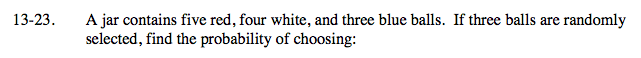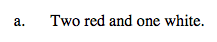$\frac{_5C_2 \cdot _4C_1}{_{12}C_5}=\frac{2}{11}$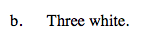See part (a).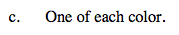See part (a).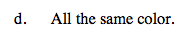$\frac{_5C_3 + _4C_3 + _3C_3}{_{12}C_3}=\frac{3}{44}$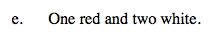See part (a).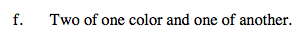How can the probabilities from parts (c) and (d)